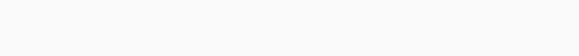# Soal Dan Kunci Jawaban Sample Paper FMO 2021 Grade 1

1. How many triangles are there in the picture?A. 7
B. 6
C. 8
D. 5
E. 9

2. Help Anna finish the picture belowA.B.C.D.E.3. Tom cut a part of a ribbon as shown below. Find the part that Tom cut?A.B.C.D.E.4. Which star contains only numbers greater than 3?
A.B.C.D.E.5. Hana is staying on the $$3^{rd}$$ floor of a 7-storey building.
How many floors are there above her?A. 4
B. 7
C. 10
D. 3
E. 2

6. John took a nap from 11am to 1pm. How many hours did he sleep?A. 2 hours
B. 3 hours
C. 4 hours
D. 5 hours
E. 1 hours

7. Fold the two corners of a rectangular paper following a dashed line. What
does the paper look like after being folded?A.B.C.D.E.8. A worker plans to build a rectangular wall. At least how many bricks are required to complete the wall?A. 7
B. 6
C. 8
D. 5
E. 9

9. Grandma has 9 gloves as below. How many pairs of gloves can she choose,
given that each pair contains 2 gloves of the same color?A. 3
B. 2
C. 1
D. 5
E. 4

10. On the way out of the maze, at least how many circles do you meet?A. 3
B. 4
C. 5
D. 6
E. 7

11. How many penguins balance the hippo?A. 5
B. 6
C. 8
D. 3
E. 2

12. Parking lot A is full. How many cars have to be moved from the left to the
right to get the same number of cars in both parking lots?A. 1
B. 4
C. 2
D. 3
E. 5

13. In a football match, the scores of teams A and B are recorded in the table
below. Each tally is considered 1 point. How many points does Team A score
more than Team B after 3 rounds?A. 7
B. 6
C. 8
D. 5
E. 4

14. Two positions of a dice are given. Which number is at the bottom?A. 1
B. 3
C. 4
D. 5
E. 6

15. How many candies do the three kids have in total?A. 10
B. 11
C. 12
D. 13
E. 14

16. During summer vacation, mother gave Mike 2 tasks to do in one week as
below.
 Task 1: Do 3 Math exercises.
 Task 2: Do 5 English exercises.
Each day, Mike must complete one of the two tasks. At the end of the first
week, Mike gave his mother 9 Math exercises. How many English exercises
did Mike do during the week?kunci jawaban: